Question

# Balance the redox equation for the oxidation of p-xylene to terephthalic acid using H2CrO4:

Balance the redox equation for the oxidation of p-xylene to terephthalic acid using H2CrO4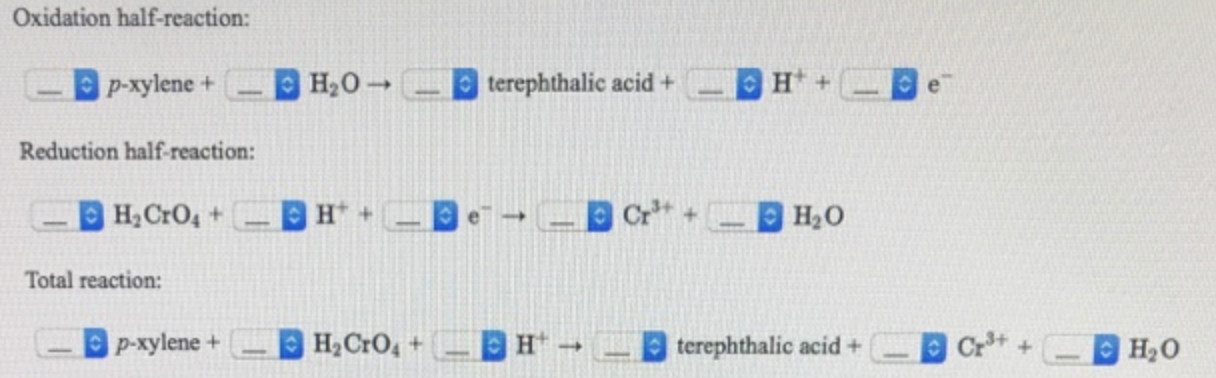How many milligrams of H2CrO4 are required to oxidize 75.0 mg of p-xylene to terephthalic acid?

Oxidation half reaction

p-xylene + H2O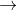terephthalic acid + 6 H+ + 6 e-

Reduction half-reaction

H2CrO4 + 6 H+ + 3 e-Cr3+ + 4 H2O

Total reaction

C8H10 + 2 H2CrO4 + 6 H+C8H6O + 2 Cr3+ + 7 H2O

milligrams of H2CrO4 = 1.67 x 103 mg (or 167 mg)

#### Earn Coins

Coins can be redeemed for fabulous gifts.

Similar Homework Help Questions
• ### balance the redox equation of: Isoborneol oxidized using Chromic Acid (H2CrO4). The products are Camphor and...

balance the redox equation of: Isoborneol oxidized using Chromic Acid (H2CrO4). The products are Camphor and Cr+3.

• ### Terephthalic acid (TPA) is synthesized from -xylene (PX) in the process C8H10 +302 – C8H6O4 +2H2O...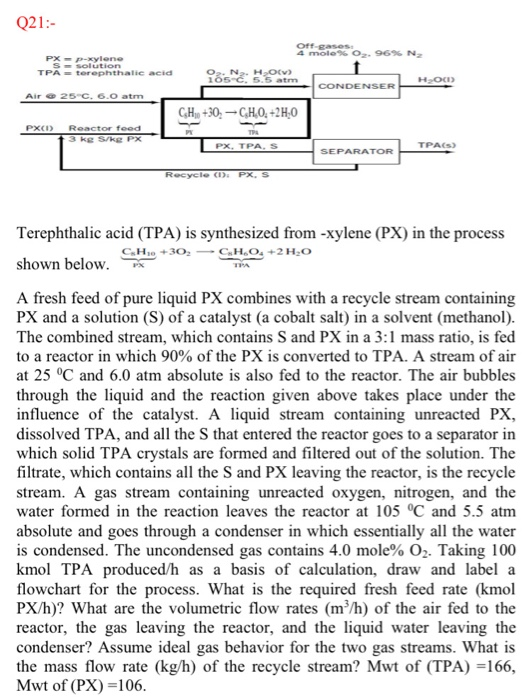Terephthalic acid (TPA) is synthesized from -xylene (PX) in the process C8H10 +302 – C8H6O4 +2H2O shown below. A fresh feed of pure liquid PX combines with a recycle stream containing PX and a solution (S) of a catalyst (a cobalt salt) in a solvent (methanol). The combined stream, which contains S and PX in a 3:1 mass ratio, is fed to a reactor in which 90% of the PX is converted to TPA. A stream of air at 25...

• ### Assignment 6.3-Balancing Redox Reactions 1 Balance the following reactions using the oxidation number method (fill in...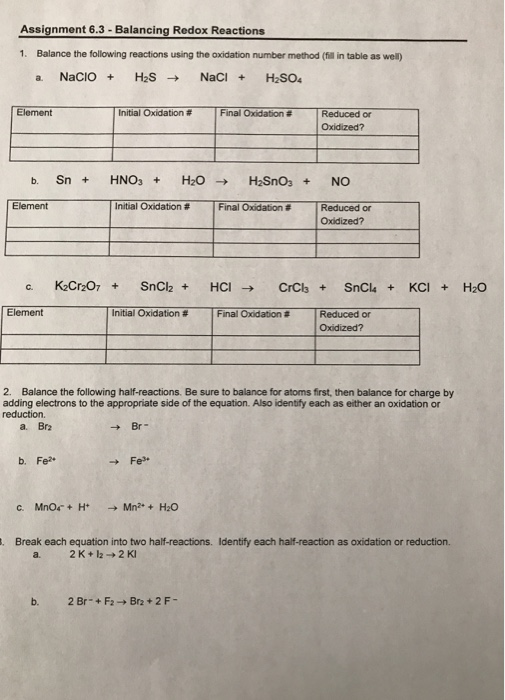Assignment 6.3-Balancing Redox Reactions 1 Balance the following reactions using the oxidation number method (fill in table as wel) a. NaClO+ H2S ? NaCl+ H2SO4 Element Initial Oxidation # Final Oxidation # Reduced or Oxidized? Element initial Oxidation # Final Oxidation # Reduced or Oxidized? K2Cr:07 + SnCl2 + HCl? CrCb + SnCl4 + KCl + H2O Element Initial Oxidation # Final Oodation # Reduced or Oxidized? 2. Balance the following half-reactions. Be sure to balance for atoms first, then...

• ### Balance them using Half Reaction method , please :) 3. Balance the following redox equation for...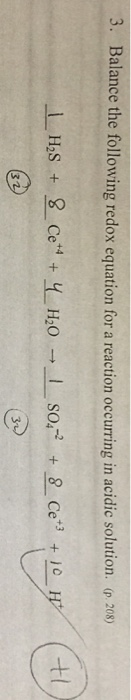Balance them using Half Reaction method , please :) 3. Balance the following redox equation for a reaction occurring in acidic solution. (p 20s Has so H20 8 Ce

• ### 5:02 17.2 Acidic Redox Reactions BALNIU ASSIGNMENT UVERVIEW 17.2 Acidic Redox Reactions Balance acidic oxidation-reduction reactions...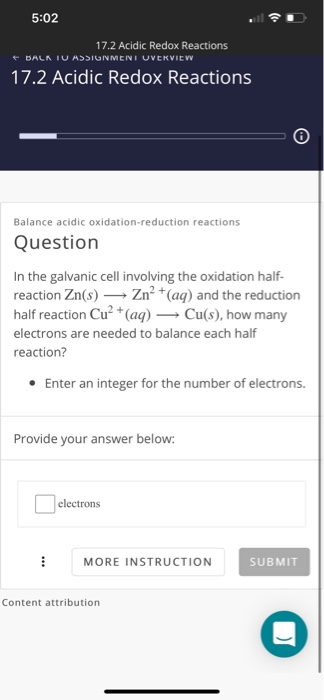5:02 17.2 Acidic Redox Reactions BALNIU ASSIGNMENT UVERVIEW 17.2 Acidic Redox Reactions Balance acidic oxidation-reduction reactions Question In the galvanic cell involving the oxidation half- reaction Zn(s) Zn²+ (aq) and the reduction half reaction Cu- (aq) — Cu(s), how many electrons are needed to balance each half reaction? • Enter an integer for the number of electrons. Provide your answer below: electrons MORE INSTRUCTION SUBMIT Content attribution

• ### Balance following equation using redox tables (acidic) (basic)

Balance following equation using redox tables (acidic) (basic)

• ### 2. Label each element with its oxidation state, use arrows to identify the reduction and oxidation, and balance the following redox reaction, which takes place under basic conditions. Please draw a b...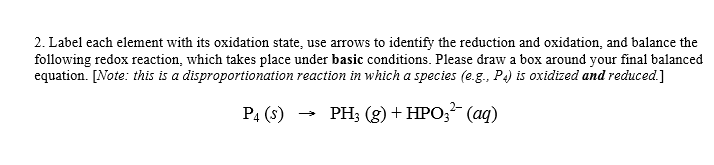2. Label each element with its oxidation state, use arrows to identify the reduction and oxidation, and balance the following redox reaction, which takes place under basic conditions. Please draw a box around your final balanced equation. [Note: this is a disproportionation reaction in which a species (e.g., P is oxidized and reduced.] P4 (s)PH (g) +HPO,2(aq) 2. Label each element with its oxidation state, use arrows to identify the reduction and oxidation, and balance the following redox reaction, which...

• ### Balance each redox reaction in acid solution. Mn2+ BiO3 MnO4 + Bi3 (acid solution) P+Cu 2+...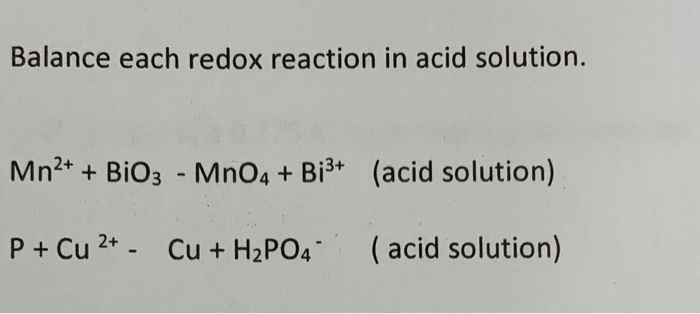Balance each redox reaction in acid solution. Mn2+ BiO3 MnO4 + Bi3 (acid solution) P+Cu 2+ - (acid solution) Cu+H2PO4

• ### Write a balanced equation for the combustion of benzoic acid, C6H3COOH, to give CO2 and H20....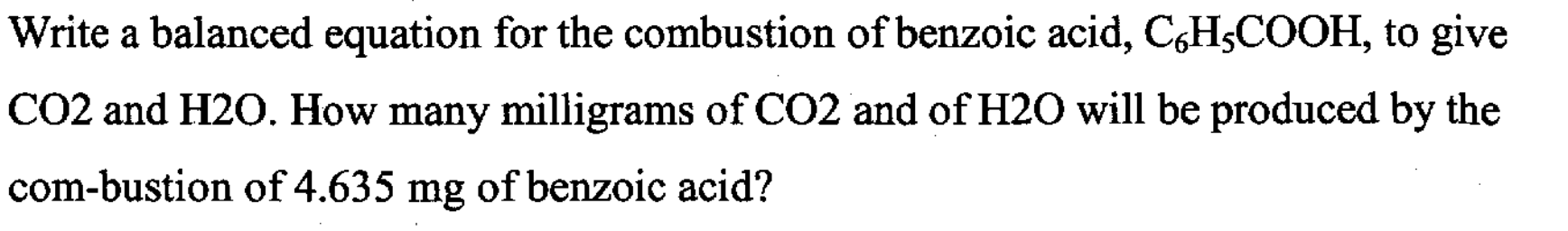Write a balanced equation for the combustion of benzoic acid, C6H3COOH, to give CO2 and H20. How many milligrams of CO2 and of H2O will be produced by the com-bustion of 4.635 mg of benzoic acid?

• ### 1.a) Balance the following redox equation in acid. In the blank put the correct stochiometric coefficient...

1.a) Balance the following redox equation in acid. In the blank put the correct stochiometric coefficient (0, 1, 2, etc.). (Note: 0 if the species does not appear on that side of the equation.) CH2O (l) + Ag+ (aq) + H2O (l) → HCO2H (aq) + Ag(s) + H+ (aq) 1.b) Balance the following redox equation in base. In the blank put the correct stochiometric coefficient (0, 1, 2, etc.). (Note: 0 if the species does not appear on that...